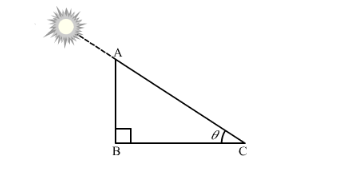# The length of shadow of a tower on the plane ground is

Question:

The length of shadow of a tower on the plane ground is $\sqrt{3}$ times the height of the tower. The angle of elevation of sun is

(a) 45°                       (b) 30°                       (c) 60°                       (d) 90°                               [CBSE 2012]

Solution:Let the angle of elevation of the sun be θ.

Suppose AB is the height of the tower and BC is the length of its shadow.

It is given that, $\mathrm{BC}=\sqrt{3} \mathrm{AB}$

In right ∆ABC,

$\tan \theta=\frac{\mathrm{AB}}{\mathrm{BC}}$

$\Rightarrow \tan \theta=\frac{\mathrm{AB}}{\sqrt{3} \mathrm{AB}}=\frac{1}{\sqrt{3}}$

$\Rightarrow \tan \theta=\tan 30^{\circ}$

$\Rightarrow \theta=30^{\circ}$

Thus, the angle of elevation of the sun is 30º.

Hence, the correct answer is option B.